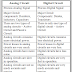The main difference between Analog Circuit and Digital Circuit is, an analog circuit is mostly built with passive elements such as resistors, inductors, and capacitors and they circulate continuous magnitude analog signals whereas a digital circuit is mostly built with active elements such as diodes, transistors and it circulates discrete magnitude digital signals. Digital circuits are more modern than analog circuits. Analog circuits and digital circuits both are used in different places.

Electronic circuits are mainly divided into these two types - analog and digital. Both of them have their own advantages, disadvantages, and working principle. An Analog signal is a continuous variable signal which means its magnitude varies with time. We measure this signal using average value, RMS value, peak value, etc. For example, an analog signal has a peak value of 10V. Here, the magnitude of the signal continuously varies up to 10V sometimes it is 5V or 7V or anything. On the other hand, a digital signal has only two values zero and its peak value. It is a discrete signal. An ideal 5V digital signal only gives you two values either 5V or 0V.

## Analog Circuit Examples

Here some common examples of Analog Circuits are,
• Audio Amplifiers
• RF Filters
• Voltage Dividers
• AC Power Circuit
• Audio Equilizer Circuit
• Noise Processing Circuit
• Operational Amplifiers
• Rectifier Circuit
• Telephone Circuitry
• Analog Computer Circuits

## Digital Circuit Examples

Here some common examples of Digital Circuits are,
• Computer Keyboard Circuit
• Digital Memory Circuit
• Digital Encoder
• Decoder
• Timer
• Counter
• Microprocessor Internal Circut
• CPU Circuit
• Remote Control Circuit
• Flip Flop
• Registers

## Difference between Analog Circuit VS Digital Circuit

 Analog Circuit Digital Circuit Process Analog Signal Process Digital Signal Major Circuit components: Resistors, Inductors, Capacitors Major Circuit Components: Diodes, Transistors There are two types of analog circuits – Active Circuits and Passive Circuits. There is only one type of digital circuit – Active Circuit. Analog circuits consume more electrical power. Digital circuits consume less electrical power. Power losses in analog circuits are very much. Power losses in digital circuits are very less. Analog circuits have more noise. Digital circuits have less noise. Analog circuits are slow in operation. Digital circuits are very fast in operation. Analog circuits are not so much accurate and precise. Digital circuits are very much accurate and precise. Analog circuit process and store signals in the form of waves. Digital Circuit processes and store signal in binary form. Analog circuits are not able to perform logical operations. Digital circuits can perform logical operations.

So, from the differences, we can see that digital circuits have so many advantages over analog circuits such as,
• Fast Operation
• Less Noise
• Less Power Loss
• Easy Design
• Compact Size
• High Efficiency
Some important applications are there where digital circuits can not be used. For example, in amplification analog signals better perform. Also, we know that when we collect data from the real world it is possible in analog signal only. So when want to process them first of all we need to convert the analog signal into a digital signal. After processing again we can convert the digital signal into an analog signal.

Analog Circuit VS Digital Circuit Examples, Use, AdvantagesReviewed by Author on October 31, 2022 Rating: 5